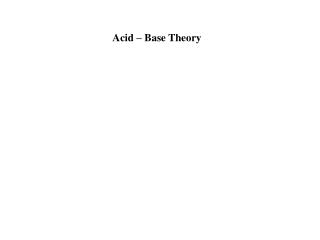DownloadDownload PresentationAcid – Base Theory

# Acid – Base Theory

Télécharger la présentation## Acid – Base Theory

- - - - - - - - - - - - - - - - - - - - - - - - - - - E N D - - - - - - - - - - - - - - - - - - - - - - - - - - -
##### Presentation Transcript

1. Acid – Base Theory

2. Definitions: Arrhenius: An acid is a substance that increases the H+ (or H3O+) concentration in an aqueous solution. HCl + H 2O  H3O+ + Cl- HCl  H+ + Cl- A base is a substance that increases the OH- concentration in an aqueous solution. NaOH(s)  Na+ + OH- What about Na2CO3 ????

3. Bronsted-Lowry: HCl(aq) + NaOH(aq) → HOH + NaCl Acid = a proton donor in a RXN Base = a proton acceptor in a RXN

4. Lewis: An acid is an electron pair acceptor H+ acid .. H:O:H .. A base is an electron pair donor water .. :O:H- ..

5. Acid/Base reactions: Produce water and a salt (and sometimes carbon dioxide). Hint: concentrate on the water first. Remember, water has the formula HOH. Complete and balance the following: HCl + KOH  HOH + KCl HCl + Ca(OH)2 2 2HOH + CaCl2 Require equal numbers

6. 1. Ba(OH)2 + H3PO4 2. HC2H3O2 + NaOH 3. H2SO4 + KOH 4. H2CO3 + NaOH 5. Na2CO3 + HCl 

7. 6. NH4OH + H2SO4 7. NH3 + HCl  Give a definition of an acid: An acid is a proton donor (H+) Give a definition of a base: A base is a proton acceptor

8. Conjugate acids and Conjugate bases HCl + KOH  HOH + KCl acid base conj. base conj. acid Na2CO3 + 2HCl  H2CO3 + 2NaCl acid conj. base base conj. acid Na2CO3 + 2HCl  H2O + CO2(g) + 2NaCl acid conj. acid base conj. base

9. NH3 + HCl  NH4+ + Cl-

10. What is a strong Acid? An Acid that is 100% ionized in water. Strong Acids: 100% ionized (completely dissociated) in water. HCl + H2O  H3O+ + Cl- often written as: HCl  H+ + Cl-

11. Strong Acids: 100% ionized (completely dissociated) in water. HCl + H2O  H3O+ + Cl- Strong Acids: Perchloric HClO4 Chloric, HClO3 Hydrobromic, HBr Hydrochloric, HCl Hydroiodic, HI Nitric, HNO3 Sulfuric, H2SO4

12. What is a strong Base? A base that is completely dissociated in water (highly soluble). NaOH(s)  Na+ + OH- Strong Bases: Group 1A metal hydroxides (LiOH, NaOH, KOH, RbOH, CsOH) Heavy Group 2A metal hydroxides [Ca(OH)2, Sr(OH)2, and Ba(OH)2]

13. Weak Acids: “The Rest”

14. Strong Acids: 100% ionized (completely dissociated) in water. HCl + H2O  H3O+ + Cl- Note the “one way arrow”. Weak Acids: Only a small % (dissociated) in water. HC2H3O2 + H2O H3O+ + C2H3O2- Note the “2-way” arrow. Why are they different?

15. Strong Acids: HCl HCl HCl HCl HCl (H2O) ADD WATER to MOLECULAR ACID

16. Strong Acids: Cl- H3O+ (H2O) Cl- H3O+ H3O+ Cl- Cl- H3O+ H3O+ Cl- Note: No HCl molecules remain in solution, all have been ionized in water.

17. Weak Acid Ionization: HC2H3O2 HC2H3O2 (H2O) HC2H3O2 HC2H3O2  HC2H3O2 Add water to MOLECULES of WEAK Acid

18. Weak Acid Ionization: HC2H3O2 HC2H3O2 H30+ C2H3O2- HC2H3O2 (H2O) HC2H3O2  H30+ C2H3O2- HC2H3O2 HC2H3O2 Note: At any given time only a small portion of the acid molecules are ionized and since reactions are running in BOTH directions the mixture composition stays the same. This gives rise to an Equilbrium expression, Ka